# COUNTIF function in Excel - count if not blank, greater than, duplicate or unique

Microsoft Excel provides several functions purposed for counting different kinds of cells, such as blanks or non-blanks, with number, date or text values, containing specific words or character, etc.

In this article, we will focus on the Excel COUNTIF function that is purposed for counting cells with the condition you specify. First, we will briefly cover the syntax and general usage, and then I provide a number of examples and warn about possible quirks when using this function with multiple criteria and specific types of cells.

In essence, COUNTIF formulas are identical in all Excel versions, so you can use the examples from this tutorial in Excel 365, 2021, 2019, 2016, 2013, 2010 and 2007.

## COUNTIF function in Excel - syntax and usage

Excel COUNTIF function is used for counting cells within a specified range that meet a certain criterion, or condition.

For example, you can write a COUNTIF formula to find out how many cells in your worksheet contain a number greater than or less than the number you specify. Another typical use of COUNTIF in Excel is for counting cells with a specific word or starting with a particular letter(s).

The syntax of the COUNTIF function is very simple:

COUNTIF(range, criteria)

As you see, there are only 2 arguments, both of which are required:

• range - defines one or several cells to count. You put the range in a formula like you usually do in Excel, e.g. A1:A20.
• criteria - defines the condition that tells the function which cells to count. It can be a number, text string, cell reference or expression. For instance, you can use the criteria like these: "10", A2, ">=10", "some text".

And here is the simplest example of Excel COUNTIF function. What you see in the image below is the list of the best tennis players for the last 14 years. The formula `=COUNTIF(C2:C15,"Roger Federer")` counts how many times Roger Federer's name is on the list: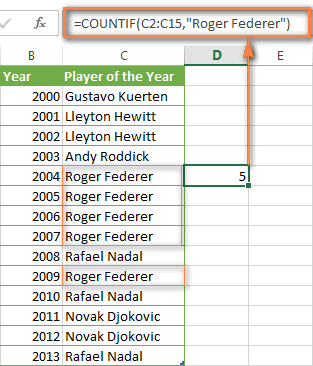Note. A criterion is case insensitive, meaning that if you type "roger federer" as the criteria in the above formula, this will produce the same result.

## Excel COUNTIF function examples

As you have just seen, the syntax of the COUNTIF function is very simple. However, it allows for many possible variations of the criteria, including wildcard characters, the values of other cells, and even other Excel functions. This diversity makes the COUNTIF function really powerful and fit for many tasks, as you will see in the examples that follow.

### COUNTIF formula for text and numbers (exact match)

In fact, we discussed the COUNTIF function that counts text values matching a specified criterion exactly a moment ago. Let me remind you that formula for cells containing an exact string of text: `=COUNTIF(C2:C15,"Roger Federer")`. So, you enter:

• A range as the first parameter;
• A comma as the delimiter;
• A word or several words enclosed in quotes as the criteria.

Instead of typing text, you can use a reference to any cell containing that word or words and get absolutely the same results, e.g. `=COUNTIF(C1:C9,C7)`.

Similarly, COUNTIF formulas work for numbers. As shown in the screenshot below, the below formula perfectly counts cells with quantity 5 in Column D:

`=COUNTIF(D2:D9, 5)`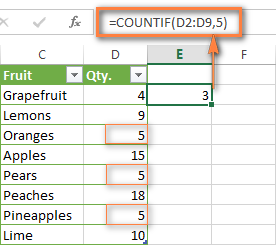In this article, you will find a few more formulas to count cells that contain any text, specific characters or only filtered cells.

### COUNTIF formulas with wildcard characters (partial match)

In case your Excel data include several variations of the keyword(s) you want to count, then you can use a wildcard character to count all the cells containing a certain word, phrase or letters as part of the cell's contents.

Suppose, you have a list of tasks assigned to different persons, and you want to know the number of tasks assigned to Danny Brown. Because Danny's name is written in several different ways, we enter "*Brown*" as the search criteria `=COUNTIF(D2:D10, "*Brown*")`.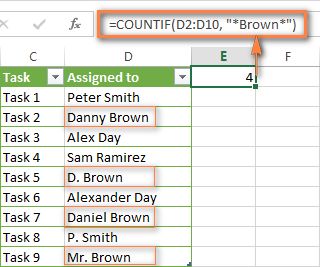An asterisk (*) is used to find cells with any sequence of leading and trailing characters, as illustrated in the above example. If you need to match any single character, enter a question mark (?) instead, as demonstrated below.

Tip. It is also possible to use wildcards with cell references with the help of the concatenation operator (&). For example, instead of supplying "*Brown*" directly in the formula, you can type it in some cell, say F1, and use the following formula to count cells containing "Brown": =COUNTIF(D2:D10, "*"&F1&"*")

#### Count cells beginning or ending with certain characters

You can use either wildcard character, asterisk (*) or question mark (?), with the criterion depending on which exactly result you want to achieve.

If you want to know the number of cells that start or end with certain text no matter how many other characters a cell contains, use these formulas:

`=COUNTIF(C2:C10,"Mr*")` - count cells that begin with "Mr".

`=COUNTIF(C2:C10,"*ed")` - count cells that end with the letters "ed".

The image below demonstrates the second formula in action: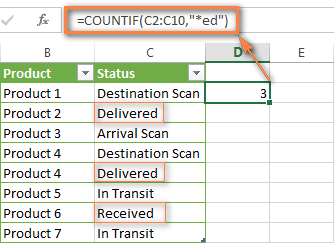If you are looking for a count of cells that start or end with certain letters and contain the exact number of characters, you use the Excel COUNTIF function with the question mark character (?) in the criteria:

`=COUNTIF(D2:D9,"??own")` - counts the number of cells ending with the letters "own" and having exactly 5 characters in cells D2 through D9, including spaces.

`=COUNTIF(D2:D9,"Mr??????")` - counts the number of cells starting with the letters "Mr" and having exactly 8 characters in cells D2 through D9, including spaces.

Tip. To find the number of cells containing an actual question mark or asterisk, type a tilde (~) before the ? or * character in the formula. For example, `=COUNTIF(D2:D9,"*~?*")` will count all cells containing the question mark in the range D2:D9.

### Excel COUNTIF for blank and non-blank cells

These formula examples demonstrate how you can use the COUNTIF function in Excel to count the number of empty or non-empty cells in a specified range.

#### COUNTIF not blank

In some Excel COUNTIF tutorials and other online resources, you may come across formulas for counting non-blank cells in Excel similar to this one:

`=COUNTIF(A1:A10,"*")`

But the fact is, the above formula counts only cells containing any text values including empty strings, meaning that cells with dates and numbers will be treated as blank cells and not included in the count!

If you need a universal COUNTIF formula for counting all non-blank cells in a specified range, here you go:

COUNTIF(range,"<>")

Or

COUNTIF(range,"<>"&"")

This formula works correctly with all value types - text, dates and numbers - as you can see in the screenshot below.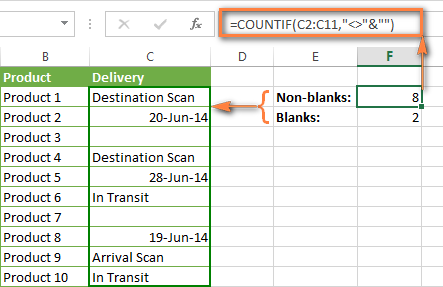#### COUNTIF blank

If you want the opposite, i.e. count blank cells in a certain range, you should adhere to the same approach - use a formula with a wildcard character for text values and with the "" criteria to count all empty cells.

Formula to count cells not containing any text:

COUNTIF(range,"<>"&"*")

Since an asterisk (*) matches any sequence of text characters, the formula counts cells not equal to *, i.e. not containing any text in the specified range.

Universal COUNTIF formula for blanks (all value types):

COUNTIF(range,"")

The above formula correctly handles numbers, dates and text values. For example, here's how you can get the number of empty cells in the range C2:C11:

`=COUNTIF(C2:C11,"")`

Please be aware that Microsoft Excel has another function for counting blank cells, COUNTBLANK. For instance, the following formulas will produce exactly the same results as the COUNTIF formulas you see in the screenshot above:

Count blanks:

`=COUNTBLANK(C2:C11)`

Count non-blanks:

`=ROWS(C2:C11)*COLUMNS(C2:C11)-COUNTBLANK(C2:C11)`

Also, please keep in mind that both COUNTIF and COUNTBLANK count cells with empty strings that only look blank. If you do not want to treat such cells as blanks, use "=" for criteria. For example:

`=COUNTIF(C2:C11,"=")`

### COUNTIF greater than, less than or equal to

To count cells with values greater than, less than or equal to the number you specify, you simply add a corresponding operator to the criteria, as shown in the table below.

Please pay attention that in COUNTIF formulas, an operator with a number are always enclosed in quotes.

Criteria Formula Example Description
Count if greater than =COUNTIF(A2:A10,">5") Count cells where value is greater than 5.
Count if less than =COUNTIF(A2:A10,"<5") Count cells with values less than 5.
Count if equal to =COUNTIF(A2:A10,"=5") Count cells where value is equal to 5.
Count if not equal to =COUNTIF(A2:A10,"<>5") Count cells where value is not equal to 5.
Count if greater than or equal to =COUNTIF(C2:C8,">=5") Count cells where value is greater than or equal to 5.
Count if less than or equal to =COUNTIF(C2:C8,"<=5") Count cells where value is less than or equal to 5.

You can also use all of the above formulas to count cells based on another cell value, you will just need to replace the number in the criteria with a cell reference.

Note. In case of a cell reference, you have to enclose the operator in quotes and add an ampersand (&) before the cell reference. For example, to count cells in the range D2:D9 with values greater than a value in cell D3, you use this formula `=COUNTIF(D2:D9,">"&D3)`: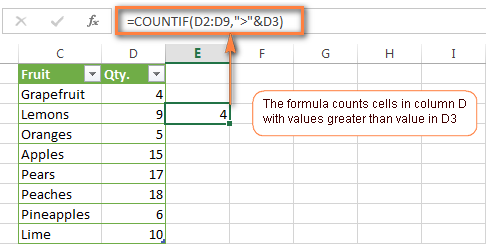If you want to count cells that contain an actual operator as part of the cell's contents, i.e. the characters ">", "<" or "=", then use a wildcard character with the operator in the criteria. Such criteria will be treated as a text string rather than a numeric expression. For example, the formula `=COUNTIF(D2:D9,"*>5*")` will count all cells in the range D2:D9 with contents like this "Delivery >5 days" or ">5 available".

### Using Excel COUNTIF function with dates

If you want to count cells with dates that are greater than, less than or equal to the date you specify or date in another cell, you proceed in the already familiar way using formulas similar to the ones we discussed a moment ago. All of the above formulas work for dates as well as for numbers. Let me give you just a few examples:

Criteria Formula Example Description
Count dates equal to the specified date. =COUNTIF(B2:B10,"6/1/2014") Counts the number of cells in the range B2:B10 with the date 1-Jun-2014.
Count dates greater than or equal to another date. =COUNTIF(B2:B10,">=6/1/2014") Count the number of cells in the range B2:B10 with a date greater than or equal to 6/1/2014.
Count dates greater than or equal to a date in another cell, minus x days. =COUNTIF(B2:B10,">="&B2-"7") Count the number of cells in the range B2:B10 with a date greater than or equal to the date in B2 minus 7 days.

Apart from these common usages, you can utilize the COUNTIF function in conjunction with specific Excel Date and Time functions such as TODAY() to count cells based on the current date.

Criteria Formula Example
Count dates equal to the current date. =COUNTIF(A2:A10,TODAY())
Count dates prior to the current date, i.e. less than today. =COUNTIF(A2:A10,"<"&TODAY())
Count dates after the current date, i.e. greater than today. =COUNTIF(A2:A10,">"&TODAY())
Count dates that are due in a week. =COUNTIF(A2:A10,"="&TODAY()+7)
Count dates in a specific date range. =COUNTIF(B2:B10, ">=6/1/2014")-COUNTIF(B2:B10, ">6/7/2014")

Here is an example of using such formulas on real data (at the moment of writing today was 25-Jun-2014):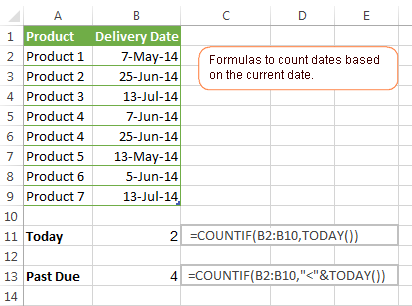### Excel COUNTIF with multiple criteria

In fact, Excel COUNTIF function is not exactly designed to count cells with multiple criteria. In most cases, you'd use its plural counterpart, the COUNTIFS function to count cells that match two or more criteria (AND logic). However, some tasks can be solved by combining two or more COUNTIF functions in one formula.

#### Count values between two numbers

One of the most common applications of Excel COUNTIF function with 2 criteria is counting numbers within a specific range, i.e. less than X but greater than Y. For example, you can use the following formula to count cells in the range B2:B9 where a value is greater than 5 and less than 15.

`=COUNTIF(B2:B9,">5")-COUNTIF(B2:B9,">=15")`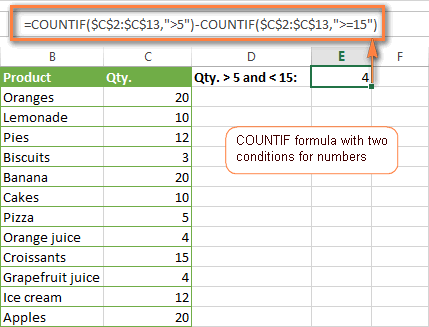How this formula works:
Here, we use two separate COUNTIF functions - the first one finds out how many values are greater than 5 and the other one gets a count of values greater than or equal to 15. Then, you subtract the latter from the former and get the desired result.

#### Count cells with multiple OR criteria

In situations when you want to get several different items in a range, add 2 or more COUNTIF functions together. Supposing, you have a shopping list and you want to find out how many soft drinks are included. To have it done, use a formula similar to this:

`=COUNTIF(B2:B13,"Lemonade")+COUNTIF(B2:B13,"*juice")`

Please pay attention that we've included the wildcard character (*) in the second criterion, it is used to count all kinds of juice on the list.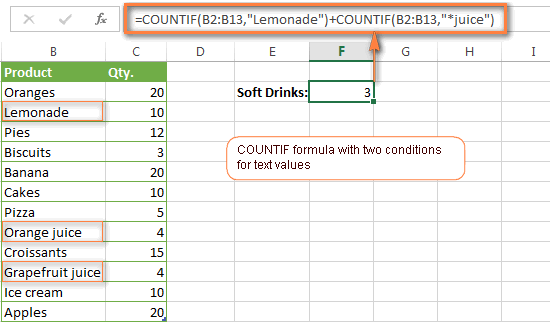In the same manner, you can write a COUNTIF formula with several conditions. Here is an example of the COUNTIF formula with multiple OR conditions that counts lemonade, juice and ice cream:

`=COUNTIF(B2:B13,"Lemonade") + COUNTIF(B2:B13,"*juice") + COUNTIF(B2:B13,"Ice cream")`

For other ways to count cells with OR logic, please see this tutorial: Excel COUNTIF and COUNTIFS with OR conditions.

### Using COUNTIF function to find duplicates and unique values

Another possible usage of the COUNTIF function in Excel is for finding duplicates in one column, between two columns, or in a row.

#### Example 1. Find and count duplicates in 1 column

For example, this simple formula =COUNTIF(B2:B10,B2)>1 will spot all duplicate entries in the range B2:B10 while another function =COUNTIF(B2:B10,TRUE) will tell you how many dupes are there: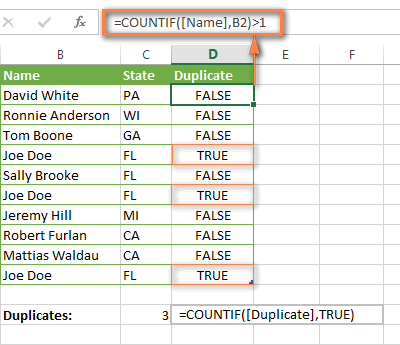#### Example 2. Count duplicates between two columns

If you have two separate lists, say lists of names in columns B and C, and you want to know how many names appear in both columns, you can use Excel COUNTIF in combination with the SUMPRODUCT function to count duplicates:

`=SUMPRODUCT((COUNTIF(B2:B1000,C2:C1000)>0)*(C2:C1000<>""))`

We can even take a step further and count how many unique names there are in Column C, i.e. names that do NOT appear in Column B:

`=SUMPRODUCT((COUNTIF(B2:B1000,C2:C1000)=0)*(C2:C1000<>""))`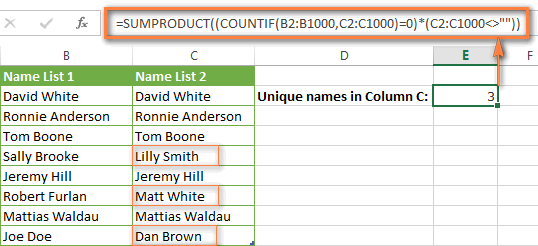Tip. If you want to highlight duplicate cells or entire rows containing duplicate entries, you can create conditional formatting rules based on the COUNTIF formulas, as demonstrated in this tutorial - Excel conditional formatting formulas to highlight duplicates.

#### Example 3. Count duplicates and unique values in a row

If you want to count duplicates or unique values in a certain row rather than a column, use one of the below formulas. These formulas might be helpful, say, to analyze the lottery draw history.

Count duplicates in a row:

`=SUMPRODUCT((COUNTIF(A2:I2,A2:I2)>1)*(A2:I2<>""))`

Count unique values in a row:

`=SUMPRODUCT((COUNTIF(A2:I2,A2:I2)=1)*(A2:I2<>""))`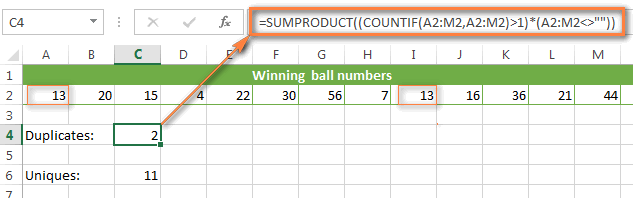## Excel COUNTIF - frequently asked questions and issues

I hope these examples have helped you to get a feel for the Excel COUNTIF function. If you've tried any of the above formulas on your data and were not able to get them to work or are having a problem with the formula you created, please look through the following 5 most common issues. There is a good chance that you will find the answer or a helpful tip there.

#### 1. COUNTIF on a non-contiguous range of cells

Question: How can I use COUNTIF in Excel on a non-contiguous range or a selection of cells?

Answer: Excel COUNTIF does not work on non-adjacent ranges, nor does its syntax allow specifying several individual cells as the first parameter. Instead, you can use a combination of several COUNTIF functions:

Wrong: `=COUNTIF(A2,B3,C4,">0")`

Right: `=COUNTIF(A2,">0") + COUNTIF(B3,">0") + COUNTIF(C4,">0")`

An alternative way is using the INDIRECT function to create an array of ranges. For example, both of the below formulas produce the same result you see in the screenshot:

`=SUM(COUNTIF(INDIRECT({"B2:B8","D2:C8"}),"=0"))`

`=COUNTIF(\$B2:\$B8,0) + COUNTIF(\$C2:\$C8,0)`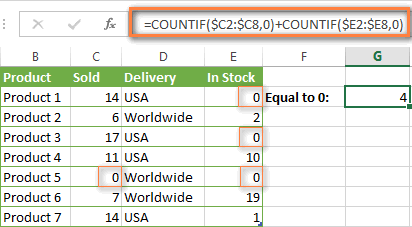#### 2. Ampersand and quotes in COUNTIF formulas

Question: When do I need to use an ampersand in a COUNTIF formula?

Answer: It is probably the trickiest part of the COUNTIF function, which I personally find very confusing. Though if you give it some thought, you'll see the reasoning behind it - an ampersand and quotes are needed to construct a text string for the argument. So, you can adhere to these rules:

If you use a number or a cell reference in the exact match criteria, you need neither ampersand nor quotes. For example:

`=COUNTIF(A1:A10,10)`
or
`=COUNTIF(A1:A10,C1)`

If your criteria includes text, wildcard character or logical operator with a number, enclose it in quotes. For example:

`=COUNTIF(A2:A10,"lemons")`
or
`=COUNTIF(A2:A10,"*")` or `=COUNTIF(A2:A10,">5")`

In case your criteria is an expression with a cell reference or another Excel function, you have to use the quotes ("") to start a text string and ampersand (&) to concatenate and finish the string off. For example:

`=COUNTIF(A2:A10,">"&D2)`
or
`=COUNTIF(A2:A10,"<="&TODAY())`

If you are in doubt whether an ampersand is needed or not, try out both ways. In most cases an ampersand works just fine, e.g. both of the below formulas work equally well.

=COUNTIF(C2:C8,"<=5")
and
=COUNTIF(C2:C8,"<="&5)

#### 3. COUNTIF for formatted (color coded) cells

Question: How do I count cells by fill or font color rather than by values?

Answer: Regrettably, the syntax of the Excel COUNTIF function does not allow using formats as the condition. The only possible way to count or sum cells based on their color is using a macro, or more precisely an Excel User-Defined function. You can find the code working for cells colored manually as well as for conditionally formatted cells in this article - How to count and sum Excel cells by fill and font color.

#### 4. #NAME? error in the COUNTIF formula

Issue: My COUNTIF formula throws a #NAME? error. How can I get it fixed?

Answer: Most likely, you have supplied an incorrect range to the formula. Please check out point 1 above.

#### 5. Excel COUNTIF formula not working

Issue: My COUNTIF formula is not working! What have I done wrong?

Answer: If you have written a formula which is seemingly correct but it does not work or produces a wrong result, start by checking the most obvious things such as a range, conditions, cell references, use of ampersand and quotes.

Be very careful with using spaces in a COUNTIF formula. When creating one of the formulas for this article I was on the verge of pulling my hair out because the correct formula (I knew with certainty it was right!) wouldn't work. As it turned out, the problem was in a measly space somewhere in between, argh... For instance, look at this formula:

`=COUNTIF(B2:B13," Lemonade")`.

At first sight, there is nothing wrong about it, except for an extra space after the opening quotation mark. Microsoft Excel will swallow the formula just fine without an error message, warning or any other indication, assuming you really want to count cells containing the word 'Lemonade' and a leading space.

If you use the COUNTIF function with multiple criteria, split the formula into several pieces and verify each function individually.

And this is all for today. In the next article, we will explore several ways to count cells in Excel with multiple conditions. Hope to see you next week and thanks for reading!

## You may also be interested in

I know that "#" stands for a number
I know that "\$" stands for a Currency
But also what sign or symbol is used for a text format?

2. Hi, I'm looking for a formula with several conditions

I have an excel master sheet where I need to create month on month graphs with over 2000 records
Column A
Reference numbers not all Unique
Column B
Creation Date (Contain Dates, no blanks)
Column V
Resolve date (contain dates and blanks)

I have been trying to get the unique count based on below conditions

2. First condition Count of anything that has a Creation date before 31/01/2023
3. Then include data where Resolve date is after 31/01/2023 and all blanks
4. Once i have that number I Need to have the count of all unique reference numbers from Column A

Your help would be much appreciated
Many Thanks